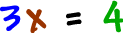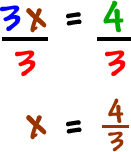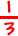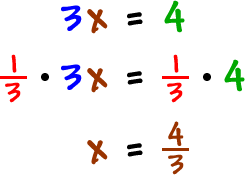We've now learned to add and subtract matrices... how to multiply a matrix by a scalar... and how to multiply two matrices...

Well, there isn't a division process for matrices.  BUT, there IS a way to get around this little problem.  We can use something we already know about: multiplication.

Here's the scoop:

Say you need to solve this guy...What do we do?  We divide both sides by 3:Let's take a closer look at what's going on here:

Instead of saying that we are dividing by 3, how about saying that we are multiplying by:The result is the same, but we used multiplication instead of division.